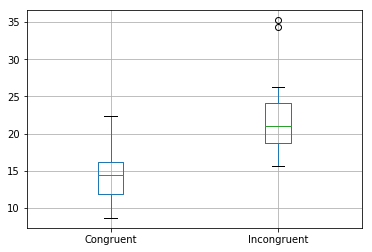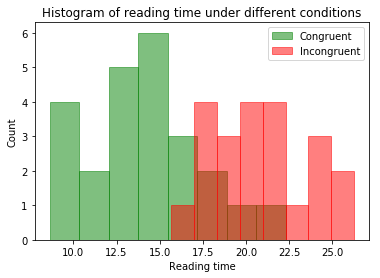### 统计学：检验心理学现象¶

(1) 我们的自变量是什么？因变量是什么？

(2) 此任务的适当假设集是什么？你需要以文字和数学符号方式对假设集中的零假设和对立假设加以说明，并对数学符号进行定义。你想执行什么类型的统计检验？为你的选择提供正当理由（比如，为何该实验满足你所选统计检验的前置条件）。

μ相同：在文字与颜色一致时，读出颜色词所花费时间的总体均值。

μ不同：在文字与颜色不一致时，读出颜色词所花费时间的总体均值。

H0：颜色不一致时，读出墨色的时间不会比一致时更多（即μ相同 ≥ μ不同）

H1：颜色不一致时，读出墨色的时间比一致时更多（即μ相同 ＜ μ不同）

(3) 报告关于此数据集的一些描述性统计。包含至少一个集中趋势测量和至少一个变异测量。

In :
# 数据集的描述性分析
import pandas as pd
import numpy as np
import matplotlib.pyplot as plt
import scipy.stats

get_ipython().run_line_magic('matplotlib', 'inline')

dfStroop.describe()

Out:
Congruent Incongruent
count
24.000000
24.000000
mean
14.051125
22.015917
std
3.559358
4.797057
min
8.630000
15.687000
25%
11.895250
18.716750
50%
14.356500
21.017500
75%
16.200750
24.051500
max
22.328000
35.255000
In :
# 异常值测量
Percentile1 = np.percentile(dfStroop['Congruent'], [0, 25, 50, 75, 100])
Percentile2 = np.percentile(dfStroop['Incongruent'], [0, 25, 50, 75, 100])

IQR = lambda p: p - p

IQR1 = IQR(Percentile1)
IQR2 = IQR(Percentile2)

up = lambda p,IQR: p + IQR*1.5
down = lambda p,IQR: p - IQR*1.5

def check(p, up, down):
count = 0
for x in p:
if (x > up) or (x < down):
count += 1
return str(count)

print('颜色一致的IQR：' + str(IQR1))
print('颜色不一致的IQR：' + str(IQR2))

up1 = float(up(Percentile1, IQR1))
up2 = float(up(Percentile2, IQR2))
down1 = float(down(Percentile1, IQR1))
down2 = float(down(Percentile2, IQR2))

print('Congruent的异常值：'+check(dfStroop['Congruent'], up1, down1)+'个')
print('Incongruent的异常值：'+check(dfStroop['Incongruent'], up2, down2)+'个')

dfStroop.boxplot();

颜色一致的IQR：4.3055

Congruent的异常值：0个
Incongruent的异常值：2个IQR：4.30（颜色一致），5.33（颜色不一致）

(4) 提供显示样本数据分布的一个或两个可视化。用一两句话说明你从图中观察到的结果。

In :
# 在这里创建可视化图表
# 清洗异常值
dfCongruent = dfStroop.loc[(dfStroop.Congruent>=down1) & (dfStroop.Congruent<=up1),'Congruent']
dfIncongruent = dfStroop.loc[(dfStroop.Incongruent>=down2) & (dfStroop.Incongruent<=up2),'Incongruent']

#绘制直方图
plt.figure()
plt.hist(dfCongruent, bins = 8,facecolor='g',edgecolor='g',alpha=0.5,label='Congruent')
plt.hist(dfIncongruent,bins = 8, facecolor='r',edgecolor='r',alpha=0.5,label='Incongruent')
plt.title('Histogram of reading time under different conditions')
plt.ylabel('Count')
plt.legend(loc=1)
plt.show()(5) 现在，执行统计测试并报告你的结果。你的置信水平和关键统计值是多少？你是否成功拒绝零假设？对试验任务得出一个结论。结果是否与你的期望一致？

In :
# 在这里执行统计检验
meanC, meanI = np.mean(dfStroop.Congruent), np.mean(dfStroop.Incongruent)
std = np.std(dfStroop.Congruent-dfStroop.Incongruent,ddof=1)
t = (meanC - meanI)/(std/np.sqrt(24))

print(t)

-8.02070694411

In :
# P值计算
p = scipy.stats.t.sf(np.abs(t), 23)

print(p)

2.05150029286e-08

In :
#置信区间计算
mean_bar = meanC - meanI
ci = std / np.sqrt(24) * 2.069

low_limit = mean_bar - ci
high_limit = mean_bar + ci
print("95%%置信区间：[%.3f , %.3f]" % (low_limit,high_limit))

95%置信区间：[-10.019 , -5.910]


In :
# 参考资源
#【如何计算IQR】https://segmentfault.com/a/1190000015926584
#【scipy.stats的ttest_rel方法】https://docs.scipy.org/doc/scipy-0.19.1/reference/generated/scipy.stats.ttest_rel.html
#【独立变量相依变量的区别？】https://github.com/ShiChJ/DAND-Basic-Materials/blob/master/P1/%E7%8B%AC%E7%AB%8B%E5%92%8C%E7%9B%B8%E4%BE%9D%E6%A0%B7%E6%9C%AC%E5%8C%BA%E5%88%AB.pdf

In [ ]: